### AME 301: Differential Equations, Vibrations and Control Homework 12

 B. GoodwineJ. Lucey Fall, 2003 Issued: December 5, 2003 Due: December 10, 2003 (US)11 December 2003 (UK)

1. Consider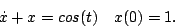1. Determine the exact solution (10 points).
2. Using Euler's method, write a computer program in any language other than a matlab to determine an approximate solution to the equation (20 points).
3. Using the fourth order Runge-Kutta method, write a computer program in any language other than matlab to determine an approximate solution to the equation (20 points).
4. In addition to submitting your work for the exact solution and a listing of each program, submit the a figure plotting the exact solution, the solution from Euler's method and the solution from the fourth order Runge-Kutta method on the same plot from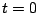to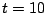(5 points each):
1.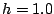2.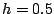3.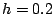4.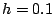2. Consider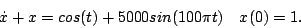1. Using Euler's method, write a computer program in any language other than a matlab to determine an approximate solution to the equation (5 points).
2. Using the fourth order Runge-Kutta method, write a computer program in any language other than matlab to determine an approximate solution to the equation (5 points).
3. In addition to submitting a listing of each program, submit a figure plotting the solution from Euler's method and the solution from the fourth order Runge-Kutta method on the same plot from t=0 to t=10 for (5 points each):
1.2.3.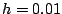4.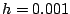4. For the same time steps (except for part (c)(i)), submit three plots where the time range is fromto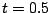(10 points total).
5. Explain what happens betweenand(20 points).

Last updated: December 5, 2003.
B. Goodwine (goodwine@controls.ame.nd.edu)
J. Lucey (jlucey@nd.edu)